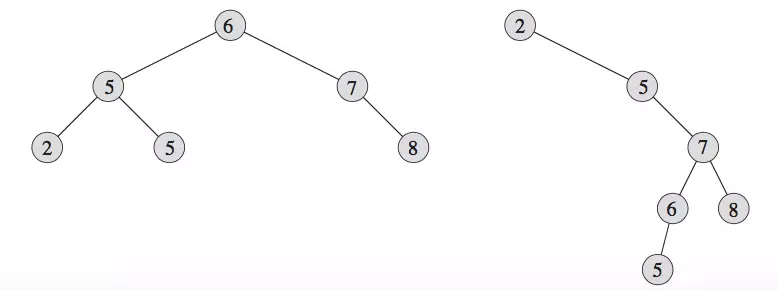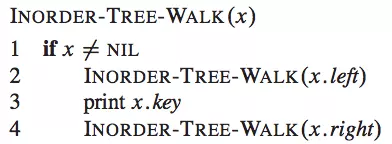# 数据结构与算法三 - 二叉搜索树

### 1 概述

二叉搜索树，顾名思义，其主要目的用于搜索，它是二叉树结构中最基本的一种数据结构，是后续理解B树、B+树、红黑树的基础，后三者在具体的工程实践中更常用，比如C++中STL就是利用红黑树做Map，B树用于磁盘上的数据库维护等，后三者均是在二叉搜索树的基础上演变而来的，理解二叉搜索树是学习后者的基础。

### 2 二叉搜索树的定义及操作

二叉树很简单，表示每个节点最多有两个子节点，二叉搜索树则更作了更近一步的要求，其必须满足如下性质：### 2.1 二叉搜索树的遍历

树的遍历有三种：先序遍历、中序遍历、后序遍历。

• 先序遍历：先访问当前节点x，再访问x的左子树x.left，最后访问x的右子树x.right
• 中序遍历：先访问当前节点x的左子树x.left, 再访问当前节点x, 最后访问x的右子树x.right
• 后序遍历：先访问当前节点x的左子树x.left, 再访问当前节点的右子树x.right, 最后访问x

中序遍历的递归算法如下：遍历二叉搜索树其实就是遍历二叉树，因为根本就没有用到二叉搜索树的特性。不过，根据二叉搜索树的特性，中序遍历得到的就是从小到大排序的结果。如果用递归算法完成遍历，其时间复杂度为:O(n)

### 2.2 二叉搜索树的查询

对于一个具有n个节点的二叉树，搜索其中某个节点x的算法的时间复杂度为O(n)，因为是n个无序节点...。而对于二叉搜索树BST，根据它的特性，其本质是一颗已排好序的节点序列，若要查找的节点元素z, 则从BST的根节点root开始查找，其算法为：

Min(t){ if t == nil return nil; if t.left == nil return t; return Min(t.left) }
Min函数的时间复杂度为O(h)，h为二叉搜索树的高度。

Max(t){ if t == nil return nil; if t.right == nil return t; return Max(t.right) }
Max函数的时间复杂度为O(h)Predecessor(t, x){ if Min(t) is x or x is nil return nil; if x.left return Max(x.left); p = x.parent; while p.right != x { x = p; p = p.parent } return p }
Predecessor算法的时间复杂度取决于Max的复杂度或while循环次数，而二者都取决于二叉搜索树的高度，所以Predecessor的时间复杂度为O(h)。Successor(t, x){ if Max(t) is x or x is nil return nil; if x.right return Min(x.right); p = x.parent; while p.left != x { x = p; p = p.parent; } return p }

### 2.3 节点插入与删除

如果采用二叉搜索树存储数据，增加和删除数据不可避免。与一般的二叉树的插入和删除不同，需要保证二叉搜索树的特性不变。

#### 2.3.1 节点插入

向二叉树中插入节点，首先面临的一个问题是，这个节点应该放在哪里？是作为叶子节点还是作为非叶子节点插入？显然，插入节点作为叶子节点是较为直接和简单的方式，问题是：是否对于任何值的节点都可以作为叶子节点插入？？？答案是肯定的，如果有兴趣可以证明（证明也比较直接），如果有怀疑，可以尝试在下图5中插入任何数值的节点，验证其二叉搜索树的特性是否成立。Insert(t, x){ while t { // while循环中查找合适的叶节点位置 y = t; if x.key < t.key t = t.left; else t = t.right; } x.parent = y if y == nil t = x; elif x.key < y.key y.left = x; else y.right = x; }
while循环的复杂度决定Insert操作的复杂度，其中的比较次数（即二叉搜索树的高度）决定了Insert的复杂度，因此其复杂度为O(h)

#### 2.3.2 删除节点

与插入节点相比，删除节点会更复杂。删除节点有两种情况：

• 删除叶节点，这种情况较为简单，直接删除不会改变二叉搜索树的特征；
• 删除非叶子节点x。这种情况稍微复杂，又可分为如下几种情况：
• 如果x的左子树或右子树为空，则直接用左子树或右子树代替x即可，如图6中的a和b图6：示例

• 如果x的左子树L和右子树均R存在，则存在两种删除的思路，一种是在左子树L中寻找最大值节点LMax（LMax比为叶子节点），删除LMax并用LMax代替x即可；另一种思路则是在右子树R中寻找最小值节点RMin（RMin必为叶子节点），删除RMin并用RMin代替x即可。

Delete(t, x) {
if t == nil
return nil;
if x.left == nil and x.right == nil {
// 叶子节点的情况
if x == t return nil;
} else if x.left != nil and x.right == nil {
// 左子树非空的情况
p = x.parent;
if x is p.left p.left = x.left;
else p.right = x.left; x.left.parent = p;
} else if x.right != nil and x.left == nil {
// 右子树非空的情况
p = x.parent;
if x is p.left p.left = x.right;
else p.right = x.right; x.right.parent = p;
} else{
//左右子树均为非空的情况
LMax = Max(x.left);
// * copy = copyAndSetNode(LMax);
// 深度复制一个LMax并对其左右子节点和父节点均赋值为nil
t = Delete(t, LMax); p = x.parent;
copy.left = x.left; copy.right = x.right;
copy.parent = p;
if x is p.left
p.left = copy;
else
p.right = copy;
}

x = nil;
return t;
}

### 3 小结

由上面的分析知：二叉搜索树的查询、插入、删除操作的时间复杂度均为O(h)。表面上看，比较理想，但是，h的值由二叉搜索树的形态决定，而二叉搜索树的形态是不确定的，如{1, 3, 5, 7, 9, 11, 13}可以构建出高度为2、3、4、5、6的二叉搜索树，这种不确定性是由于二叉搜索树限定的特征较为宽松造成的。在二叉搜索树上加上其它限定特征，即可构成B树、B+树、红黑树等。

posted @ 2019-11-06 19:57  TANZAME  阅读(120)  评论(0编辑  收藏